Search by Topic

Resources tagged with Addition & subtraction similar to Remainders:

Filter by: Content type:
Age range:
Challenge level:

There are 215 resultsBeing Resilient - Primary Number

Age 5 to 11 Challenge Level:

Number problems at primary level that may require resilience.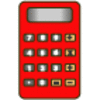Clever Keys

Age 7 to 11 Short Challenge Level:

On a calculator, make 15 by using only the 2 key and any of the four operations keys. How many ways can you find to do it?Being Collaborative - Primary Number

Age 5 to 11 Challenge Level:

Number problems at primary level to work on with others.Zios and Zepts

Age 7 to 11 Challenge Level:

On the planet Vuv there are two sorts of creatures. The Zios have 3 legs and the Zepts have 7 legs. The great planetary explorer Nico counted 52 legs. How many Zios and how many Zepts were there?Book Codes

Age 7 to 11 Challenge Level:

Look on the back of any modern book and you will find an ISBN code. Take this code and calculate this sum in the way shown. Can you see what the answers always have in common?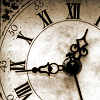Clock Face

Age 7 to 11 Challenge Level:

Where can you draw a line on a clock face so that the numbers on both sides have the same total?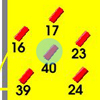Throw a 100

Age 7 to 11 Challenge Level:

Can you score 100 by throwing rings on this board? Is there more than way to do it?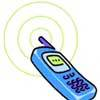Mobile Numbers

Age 5 to 11 Challenge Level:

In this investigation, you are challenged to make mobile phone numbers which are easy to remember. What happens if you make a sequence adding 2 each time?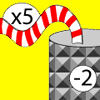Function Machines

Age 7 to 11 Challenge Level:

If the numbers 5, 7 and 4 go into this function machine, what numbers will come out?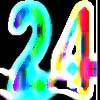Special 24

Age 7 to 11 Challenge Level:

Find another number that is one short of a square number and when you double it and add 1, the result is also a square number.The 24 Game

Age 7 to 11 Challenge Level:

There are over sixty different ways of making 24 by adding, subtracting, multiplying and dividing all four numbers 4, 6, 6 and 8 (using each number only once). How many can you find?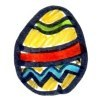How Many Eggs?

Age 7 to 11 Challenge Level:

Peter, Melanie, Amil and Jack received a total of 38 chocolate eggs. Use the information to work out how many eggs each person had.As Easy as 1,2,3

Age 11 to 14 Challenge Level:

When I type a sequence of letters my calculator gives the product of all the numbers in the corresponding memories. What numbers should I store so that when I type 'ONE' it returns 1, and when I type. . . .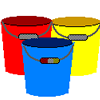Buckets of Thinking

Age 7 to 11 Challenge Level:

There are three buckets each of which holds a maximum of 5 litres. Use the clues to work out how much liquid there is in each bucket.Sam's Quick Sum

Age 7 to 11 Challenge Level:

What is the sum of all the three digit whole numbers?The Clockmaker's Birthday Cake

Age 7 to 11 Challenge Level:

The clockmaker's wife cut up his birthday cake to look like a clock face. Can you work out who received each piece?Numbers Numbers Everywhere!

Age 5 to 11

Bernard Bagnall recommends some primary school problems which use numbers from the environment around us, from clocks to house numbers.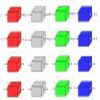Machines

Age 7 to 11 Challenge Level:

What is happening at each box in these machines?Scoring with Dice

Age 7 to 11 Challenge Level:

I throw three dice and get 5, 3 and 2. Add the scores on the three dice. What do you get? Now multiply the scores. What do you notice?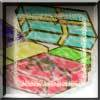Age 7 to 11 Challenge Level:

Use the information to work out how many gifts there are in each pile.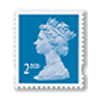Penta Post

Age 7 to 11 Challenge Level:

Here are the prices for 1st and 2nd class mail within the UK. You have an unlimited number of each of these stamps. Which stamps would you need to post a parcel weighing 825g?3388

Age 11 to 14 Challenge Level:

Using some or all of the operations of addition, subtraction, multiplication and division and using the digits 3, 3, 8 and 8 each once and only once make an expression equal to 24.Napier's Bones

Age 7 to 11 Challenge Level:

The Scot, John Napier, invented these strips about 400 years ago to help calculate multiplication and division. Can you work out how to use Napier's bones to find the answer to these multiplications?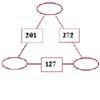Number Juggle

Age 7 to 11 Challenge Level:

Fill in the missing numbers so that adding each pair of corner numbers gives you the number between them (in the box).Making Pathways

Age 7 to 11 Challenge Level:

Can you find different ways of creating paths using these paving slabs?Abundant Numbers

Age 7 to 11 Challenge Level:

48 is called an abundant number because it is less than the sum of its factors (without itself). Can you find some more abundant numbers?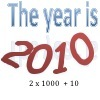It Was 2010!

Age 5 to 11 Challenge Level:

If the answer's 2010, what could the question be?Arrange the Digits

Age 11 to 14 Challenge Level:

Can you arrange the digits 1,2,3,4,5,6,7,8,9 into three 3-digit numbers such that their total is close to 1500?The Deca Tree

Age 7 to 11 Challenge Level:

Find out what a Deca Tree is and then work out how many leaves there will be after the woodcutter has cut off a trunk, a branch, a twig and a leaf.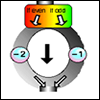The Number Crunching Machine

Age 7 to 11 Challenge Level:

Put a number at the top of the machine and collect a number at the bottom. What do you get? Which numbers get back to themselves?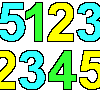Sept03 Sept03 Sept03

Age 7 to 11 Challenge Level:

This number has 903 digits. What is the sum of all 903 digits?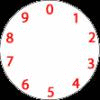Dividing a Cake

Age 7 to 11 Challenge Level:

Annie cut this numbered cake into 3 pieces with 3 cuts so that the numbers on each piece added to the same total. Where were the cuts and what fraction of the whole cake was each piece?I'm Eight

Age 5 to 11 Challenge Level:

Find a great variety of ways of asking questions which make 8.Reverse Trick

Age 7 to 11 Challenge Level:

Tell your friends that you have a strange calculator that turns numbers backwards. What secret number do you have to enter to make 141 414 turn around?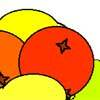Oranges and Lemons

Age 7 to 11 Challenge Level:

On the table there is a pile of oranges and lemons that weighs exactly one kilogram. Using the information, can you work out how many lemons there are?Six Is the Sum

Age 7 to 11 Challenge Level:

What do the digits in the number fifteen add up to? How many other numbers have digits with the same total but no zeros?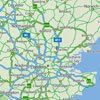Journeys

Age 7 to 11 Challenge Level:

Investigate the different distances of these car journeys and find out how long they take.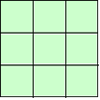1, 2, 3 Magic Square

Age 7 to 11 Challenge Level:

Arrange three 1s, three 2s and three 3s in this square so that every row, column and diagonal adds to the same total.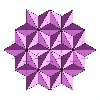Next Number

Age 7 to 11 Short Challenge Level:

Find the next number in this pattern: 3, 7, 19, 55 ...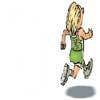Rocco's Race

Age 7 to 11 Short Challenge Level:

Rocco ran in a 200 m race for his class. Use the information to find out how many runners there were in the race and what Rocco's finishing position was.Calendar Calculations

Age 7 to 11 Challenge Level:

Try adding together the dates of all the days in one week. Now multiply the first date by 7 and add 21. Can you explain what happens?Exploring Wild & Wonderful Number Patterns

Age 7 to 11 Challenge Level:

EWWNP means Exploring Wild and Wonderful Number Patterns Created by Yourself! Investigate what happens if we create number patterns using some simple rules.Escape from the Castle

Age 7 to 11 Challenge Level:

Skippy and Anna are locked in a room in a large castle. The key to that room, and all the other rooms, is a number. The numbers are locked away in a problem. Can you help them to get out?Sometimes We Lose Things

Age 7 to 11 Challenge Level:

Well now, what would happen if we lost all the nines in our number system? Have a go at writing the numbers out in this way and have a look at the multiplications table.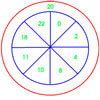On Target

Age 7 to 11 Challenge Level:

You have 5 darts and your target score is 44. How many different ways could you score 44?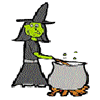Hubble, Bubble

Age 7 to 11 Challenge Level:

Winifred Wytsh bought a box each of jelly babies, milk jelly bears, yellow jelly bees and jelly belly beans. In how many different ways could she make a jolly jelly feast with 32 legs?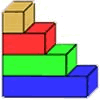Rod Measures

Age 7 to 11 Challenge Level:

Using 3 rods of integer lengths, none longer than 10 units and not using any rod more than once, you can measure all the lengths in whole units from 1 to 10 units. How many ways can you do this?A Mixed-up Clock

Age 7 to 11 Challenge Level:

There is a clock-face where the numbers have become all mixed up. Can you find out where all the numbers have got to from these ten statements?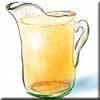Pouring the Punch Drink

Age 7 to 11 Challenge Level:

There are 4 jugs which hold 9 litres, 7 litres, 4 litres and 2 litres. Find a way to pour 9 litres of drink from one jug to another until you are left with exactly 3 litres in three of the jugs.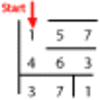Maze 100

Age 7 to 11 Challenge Level:

Can you go through this maze so that the numbers you pass add to exactly 100?